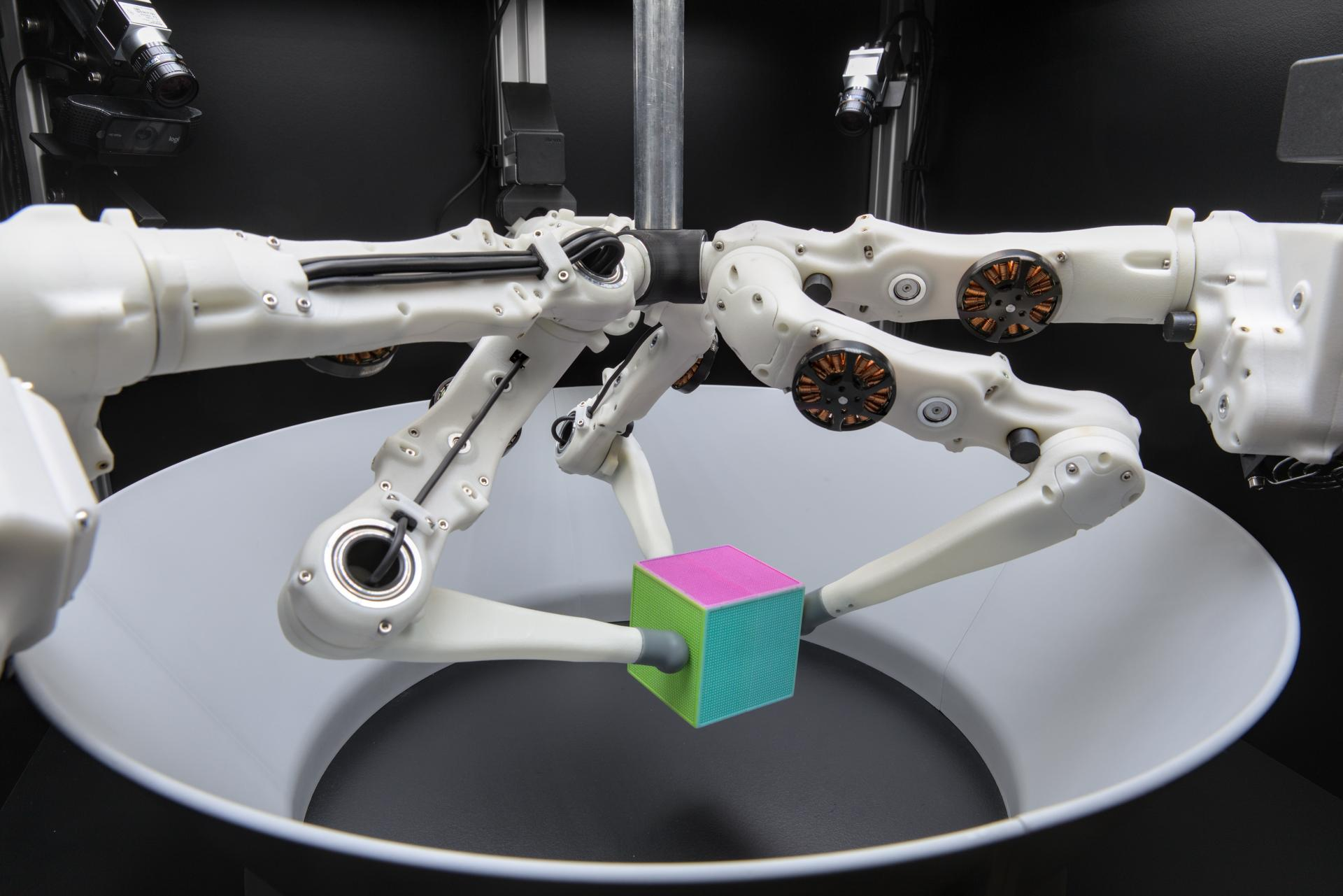Task 1: Move Cube on Trajectory¶The goal of this task is to grasp a cube and move it from one goal to the next on a given trajectory.

The trajectory is given as a list of tuples (t, goal_position) where t specifies the time step from which the goal position is active. So the full type is Sequence[Tuple[int, Sequence[float]]]

Example:

trajectory = [
(0, (0, 0, 0.0325)),
(4000, (0, 0.05, 0.0325)),
(8000, (0.05, 0.05, 0.0325)),
]

The first goal (0, 0, 0.0325) is active at the beginning. Starting from time step 4000 the active goal switches to (0, 0.05, 0.0325). At step 8000 (0.05, 0.05, 0.0325) becomes the active goal and stays active until the end of the run.

The duration of a run is 120000 steps (~2 minutes). This value is also given by EPISODE_LENGTH.

The cost of each step is computed using the “move cube to goal” cost of the currently active goal (see trifinger_simulation.tasks.move_cube.evaluate_state(), using difficulty 3).

Duration of the episode in time steps (corresponds to ~2 minutes).

Sample a goal trajectory with random steps.

The number of goals in the trajectory is depending on the episode length (see EPISODE_LENGTH). The first goal has a duration of FIRST_GOAL_DURATION steps, the following ones a duration of GOAL_DURATION.

Returns

Trajectory as a list of tuples (t, position) where t marks the time step from which the goal is active.

Return type

Sequence[Tuple[int, Sequence[float]]]

Compute cost of a given cube pose at a given time step. Less is better.

As cost the position error between actual_position and the trajectory goal that is active at the given time_index is used. It is computed as a weighted sum of the Euclidean distance on the x/y-plane and the absolute distance on the z-axis. Both components are scaled based on their expected range. Since the z-range is smaller, this means that the height has a higher weight. The sum is again rescaled so that the total error is in the interval [0, 1].

Input: active goal position and actual cube position

err_xy = ||goal[xy] - actual[xy]||
err_z  = |goal[z] - actual[z]|

cost = (err_xy / arena_diameter + err_z / height_range) / 2
Parameters
• trajectory (Sequence[Tuple[int, Sequence[float]]]) – The trajectory based on which the cost is computed.

• time_index (int) – Index of the time step that is evaluated.

• actual_position (Sequence[float]) – Cube position at the specified time step.

Returns

Cost of the actual position w.r.t. to the goal position of the active step in the trajectory. Lower value means that the actual pose is closer to the goal. Zero if actual == goal.

Get the trajectory goal that is active at the given time step.

Parameters
• trajectory (Sequence[Tuple[int, Sequence[float]]]) – The trajectory.

• time_index (int) – Index of the desired time step.

Returns

The goal from the given trajectory that is active at the given time step.

Return type

Optional[Sequence[float]]

Checks if the given trajectory is valid, raises error if not.

Raises
• ValueError – If there are any structural issues.

• move_cube.InvalidGoalError – If a position exceeds the allowed goal space.

Parameters

trajectory (Sequence[Tuple[int, Sequence[float]]]) –

Load or sample a goal based on the given goal config file.

Parameters

filename (str) – Path to the goal config JSON file. If it contains an entry “goal”, its value is used as goal. Otherwise a random goal is sampled.

Returns

The goal as JSON-encoded string.

Return type

str